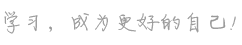## n是什么意思

1.字母n
abbr.
1.=[Lat] natus (=born)
2.=[Commerce] net
3.=noon
4.=note
5.=noun
6.=number
N.
abbr.
1.=Norse
2.=November

• the cardinal compass point that is at 0 or 360 degrees
• the 14th letter of the Roman alphabet
• a unit of force equal to the force that imparts an acceleration of 1 m/sec/sec to a mass of 1 kilogram; equal to 100,000 dynes
• a common nonmetallic element that is normally a colorless odorless tasteless inert diatomic gas; constitutes 78 percent of the atmosphere by volume; a constituent of all living tissues
• (of a solution) concentration expressed in gram equivalents of solute per liter

• n n. 1.字母n abbr. 1.=[Lat] natus (=born) 2.=[Commerce] net 3.=noon 4.=note 5.=noun 6.=number
• 'n con.=and;=than
• n =-en
• N =-en
• N n.字母n
• n/n 【缩写】 =not to be noted 【商】未记帐,不作公证人记录;不注意
• N/N 非流通；不可转让 non-negotiable,not negotiable.
• N.N.R. 【医】 非法定新药集
• N,N dimethylaniline N,N-二甲基苯胺
• N,N dimethyltryptamine 【化】 N,N-二甲基色胺